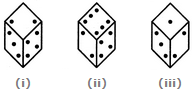# Non Verbal Reasoning - Cubes and Dice - Discussion

### Discussion :: Cubes and Dice - Section 2 (Q.No.37)

37.

Given below are three different positions of a dice. Find the number of dots on the face opposite the face bearing 3 dots.[A]. 4 [B]. 5 [C]. 6 [D]. Cannot be determined

Explanation:

From figures (i), (ii) and (iii), we conclude that 3, 4, 6 and 1 dots appear adjacent to 2 dots. Therefore, 5 dots appear opposite 2 dots. Now, from figures (i) and (iii), we conclude that 2, 4 and 1 dots appear adjacent to 3 dots. Therefore, either 5 or 6 dots appear opposite 3 dots. Since, 5 dots appear opposite 2 dots, it follows that 6 dots appear opposite 3 dots.

 Simon said: (Jun 24, 2016) If you calculate like in the explanation it's correct but if you turn the first dice in the position of the second. The 4 won't be at the same place like in the 2nd Picture. That means that dice one and dice two isn't the same dice. That would mean the answer is D: Cannot be determined.